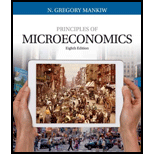Chapter 12, Problem 3CQQ### Principles of Microeconomics (Mind...

8th Edition
N. Gregory Mankiw
ISBN: 9781305971493

#### Solutions

Chapter
Section### Principles of Microeconomics (Mind...

8th Edition
N. Gregory Mankiw
ISBN: 9781305971493
Textbook Problem

# If the tax code exempts the first $20,000 of income from taxation and then taxes 25 percent of all income above that level, then a person who earns$50,000 has an average tax rate of _______ percent and a marginal tax rate of _______ percent.a. 15, 25b. 25. 15c. 25, 30d. 30. 25

To determine

The average tax rate and marginal tax rate.

Explanation

The first $20,000 of the income is exempted from the taxation and then 25% tax is levied on all the income above that level. The income of a person is$50,000.

Option (a):

Calculation of average tax rate:

Average tax rate =(Total incomeTax exemption)×Tax rateTotal income=(

### Still sussing out bartleby?

Check out a sample textbook solution.

See a sample solution

#### The Solution to Your Study Problems

Bartleby provides explanations to thousands of textbook problems written by our experts, many with advanced degrees!

Get Started